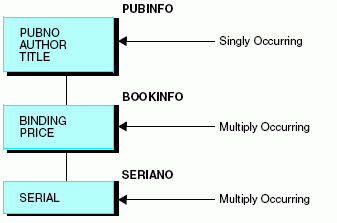# Describing a Multiply Occurring Field in a Free-Format Data Source

Since any data field not explicitly referred to in a logical record continues to have the same value as it did the last time one was assigned, up until the point a new data value is entered, a free-format sequential data source can resemble a hierarchical structure. The parent information needs to be entered only once, and it carries over for each descendant segment.

## Example: Describing a Multiply Occurring Field in a Free-Format Data Source

Consider the example of a library data source. The information for two copies of The Sun Also Rises, one hardcover and one paperback, can be entered as follows:

```PUBNO=1234567890, AUTHOR='Hemingway, Ernest',
TITLE='The Sun Also Rises',
BI=H,PR=17.95, \$
BI=S,PR=5.25, \$```

There are two values for binding and price, which both correspond to the same publisher number, author, and title. In the Master File, the information that occurs only once (the publisher number, author, and title) is placed in one segment, and the information that occurs several times in relation to this information is placed in a descendant segment.

Similarly, information that occurs several times in relation to the descendant segment, such as an individual serial number for each copy of the book, is placed in a segment that is a descendant of the first descendant segment, as shown in the following diagram:Describe this data source as follows:

```FILENAME = LIBRARY4, SUFFIX = COM, \$
SEGNAME = PUBINFO, SEGTYPE=S0, \$
FIELDNAME = PUBNO,   ALIAS = PN,   USAGE = A10,   ACTUAL = A10, \$
FIELDNAME = AUTHOR,  ALIAS = AT,   USAGE = A25,   ACTUAL = A25, \$
FIELDNAME = TITLE,   ALIAS = TL,   USAGE = A50,   ACTUAL = A50, \$
SEGNAME = BOOKINFO, PARENT = PUBINFO,  SEGTYPE=S0, \$
FIELDNAME = BINDING, ALIAS = BI,   USAGE = A1,    ACTUAL = A1,  \$
FIELDNAME = PRICE,   ALIAS = PR,   USAGE = D8.2N, ACTUAL = D8,  \$
SEGNAME = SERIANO,  PARENT = BOOKINFO, SEGTYPE=S0, \$
FIELDNAME = SERIAL,  ALIAS = SN,   USAGE = A15,   ACTUAL = A15, \$```

Note that each segment other than the first has a PARENT attribute. You use the PARENT attribute to signal that you are describing a hierarchical structure.

 WebFOCUS